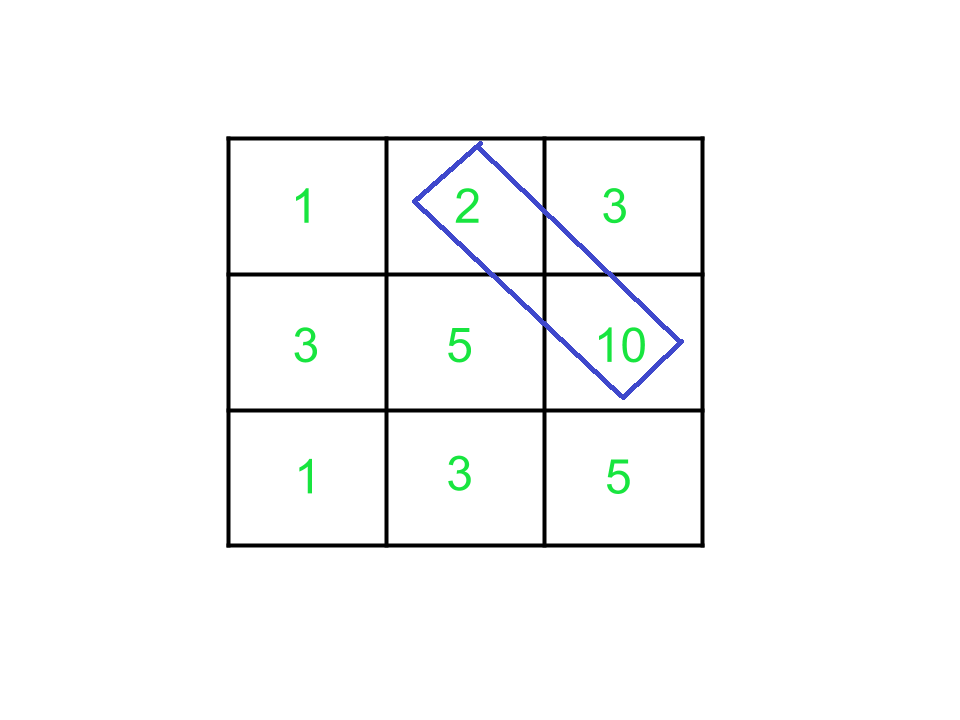# Maximize sum by traversing diagonally from each cell of a given Matrix

• Last Updated : 29 May, 2021

Given a 2D square matrix arr[][] of dimensions N x N, the task is to find the maximum path sum by moving diagonally from any cell and each cell must be visited only once i.e., from the cell (i, j), a player can move to the cell (i + 1, j + 1).Examples:

Input: arr[][] = {{1, 2, 3}, {3, 5, 10}, {1 3 5}}
Output: 12
Explanation:
Sum of cells (1, 1), (2, 2) and (3, 3) is 11.
The sum of cells (1, 2), (2, 3) and (1, 3) is 3.
The sum of cells (2, 1) and (3, 2) is 6.
The sum of cell (3, 1) is 1.
The maximum possible sum is 12.

Input: arr[][] = {{1, 1, 1}, {1 1 1}, {1 1 1}}
Output: 3

Approach: To solve this problem, the idea is to traverse the matrix diagonally for first row and column elements and sum up their diagonal elements within the range of the matrix.
Follow the steps below to solve the problem:

1. Initialize a variable, say max with 0.
2. Choose each cell (i, j) from the first row and from the first column.
3. Now, from each cell, find the diagonal sum starting from that cell by incrementing i and j by 1, say sum.
4. Then, update max as max(max, sum).
5. After traversing, print max as the required answer.

Below is the implementation of the above approach:

## C++

 `// C++ program for the above approach` `#include ``using` `namespace` `std;` `// Function to find the maximum sum``int` `MaximumSum(vector >& arr, ``int` `n)``{` `    ``int` `ans = 0;` `    ``// Loop to traverse through the``    ``// upper triangular matrix and``    ``// update the maximum sum to ans``    ``for` `(``int` `i = 0; i < n; i++) {``        ``int` `x = 0, y = i, sum = 0;``        ``for` `(``int` `j = i; j < n; j++) {``            ``sum += arr[x++][y++];``        ``}``        ``if` `(sum > ans)``            ``ans = sum;``    ``}` `    ``// Traverse through the``    ``// lower triangular matrix``    ``for` `(``int` `i = 1; i < n; i++) {` `        ``int` `x = i, y = 0, sum = 0;` `        ``for` `(``int` `j = i; j < n; j++) {` `            ``sum += arr[x++][y++];``        ``}``        ``if` `(sum > ans)``            ``ans = sum;``    ``}` `    ``return` `ans;``}` `// Driver Code``int` `main()``{` `    ``// Given matrix``    ``vector > arr;``    ``arr = { { 1, 2, 3 },``            ``{ 3, 5, 10 },``            ``{ 1, 3, 5 } };` `    ``// Given dimension``    ``int` `n = arr.size();` `    ``cout << MaximumSum(arr, n);` `    ``return` `0;``}`

## Java

 `// Java program for the above approach``import` `java.util.*;``class` `GFG{` `// Function to find the maximum sum``static` `int` `MaximumSum(``int` `[][]arr, ``int` `n)``{` `    ``int` `ans = ``0``;` `    ``// Loop to traverse through the``    ``// upper triangular matrix and``    ``// update the maximum sum to ans``    ``for` `(``int` `i = ``0``; i < n; i++)``    ``{``        ``int` `x = ``0``, y = i, sum = ``0``;``        ``for` `(``int` `j = i; j < n; j++)``        ``{``            ``sum += arr[x++][y++];``        ``}``        ``if` `(sum > ans)``            ``ans = sum;``    ``}` `    ``// Traverse through the``    ``// lower triangular matrix``    ``for` `(``int` `i = ``1``; i < n; i++)``    ``{``        ``int` `x = i, y = ``0``, sum = ``0``;``        ``for` `(``int` `j = i; j < n; j++)``        ``{``            ``sum += arr[x++][y++];``        ``}``        ``if` `(sum > ans)``            ``ans = sum;``    ``}``    ``return` `ans;``}` `// Driver Code``public` `static` `void` `main(String[] args)``{` `    ``// Given matrix``    ``int` `[][]arr = { { ``1``, ``2``, ``3` `},``            ``{ ``3``, ``5``, ``10` `},``            ``{ ``1``, ``3``, ``5` `} };` `    ``// Given dimension``    ``int` `n = arr.length;``    ``System.out.print(MaximumSum(arr, n));``}``}` `// This code is contributed by 29AjayKumar`

## Python3

 `# Python3 program for the above approach` `# Function to find the maximum sum``def` `MaximumSum(arr, n):``    ``ans ``=` `0``;` `    ``# Loop to traverse through the``    ``# upper triangular matrix and``    ``# update the maximum sum to ans``    ``for` `i ``in` `range``(n):``        ``x, y, ``sum` `=` `0``, i, ``0``        ``for` `j ``in` `range``(i, n):``            ``sum``, x, y ``=``sum` `+` `arr[x][y], x ``+` `1``, y ``+` `1``        ``if` `(``sum` `> ans):``            ``ans ``=` `sum` `    ``# Traverse through the``    ``# lower triangular matrix``    ``for` `i ``in` `range``(``1``, n):` `        ``x, y, ``sum` `=` `i, ``0``, ``0` `        ``for` `j ``in` `range``(i, n):` `            ``sum``, x, y ``=``sum` `+` `arr[x][y], x ``+` `1``, y ``+` `1``        ``if` `(``sum` `> ans):``            ``ans ``=` `sum``    ``return` `ans` `# Driver Code``if` `__name__ ``=``=` `'__main__'``:` `    ``# Given matrix``    ``arr ``=` `[ [ ``1``, ``2``, ``3``],``            ``[ ``3``, ``5``, ``10``],``            ``[ ``1``, ``3``, ``5` `]]` `    ``# Given dimension``    ``n ``=` `len``(arr)``    ``print` `(MaximumSum(arr, n))` `    ``# This code is contributed by mohit kumar 29`

## C#

 `// C# program for the above approach``using` `System;``class` `GFG{` `  ``// Function to find the maximum sum``  ``static` `int` `MaximumSum(``int` `[,]arr, ``int` `n)``  ``{``    ``int` `ans = 0;` `    ``// Loop to traverse through the``    ``// upper triangular matrix and``    ``// update the maximum sum to ans``    ``for` `(``int` `i = 0; i < n; i++)``    ``{``      ``int` `x = 0, y = i, sum = 0;``      ``for` `(``int` `j = i; j < n; j++)``      ``{``        ``sum += arr[x++, y++];``      ``}``      ``if` `(sum > ans)``        ``ans = sum;``    ``}` `    ``// Traverse through the``    ``// lower triangular matrix``    ``for` `(``int` `i = 1; i < n; i++)``    ``{``      ``int` `x = i, y = 0, sum = 0;``      ``for` `(``int` `j = i; j < n; j++)``      ``{``        ``sum += arr[x++, y++];``      ``}``      ``if` `(sum > ans)``        ``ans = sum;``    ``}``    ``return` `ans;``  ``}` `  ``// Driver Code``  ``public` `static` `void` `Main(String[] args)``  ``{` `    ``// Given matrix``    ``int` `[,]arr = { { 1, 2, 3 },``                  ``{ 3, 5, 10 },``                  ``{ 1, 3, 5 } };` `    ``// Given dimension``    ``int` `n = arr.GetLength(0);``    ``Console.Write(MaximumSum(arr, n));``  ``}``}` `// This code is contributed by shikhasingrajput`

## Javascript

 ``

Output:

`12`

Time Complexity: O(N2)
Auxiliary Space: O(N2)

My Personal Notes arrow_drop_up